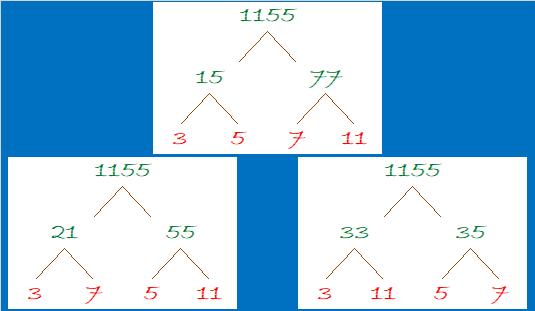# Reasons to Celebrate 1155

1155 is the product of the four smallest odd prime numbers. That alone is a reason to celebrate. Here, I’ve made a factor cake with 1155 for you to enjoy!make science GIFs like this at MakeaGif

Besides 1155 being the smallest number divisible by the first 4 odd prime numbers, what else can I say about it?

• 1155 is a composite number.
• Prime factorization: 1155 = 3 × 5 × 7 × 11
• The exponents in the prime factorization are 1, 1, 1, and 1. Adding one to each and multiplying we get (1 + 1)(1 + 1)(1 + 1)(1 + 1) = 2 × 2 × 2 × 2 = 16. Therefore 1155 has exactly 16 factors.
• Factors of 1155: 1, 3, 5, 7, 11, 15, 21, 33, 35, 55, 77, 105, 165, 231, 385, 1155
• Factor pairs: 1155 = 1 × 1155, 3 × 385, 5 × 231, 7 × 165, 11 × 105, 15 × 77, 21 × 55, or 33 × 35
• 1155 has no square factors that allow its square root to be simplified. √1155 ≈ 33.98529Since it’s number of prime factors is a power of 2, it can make some very well-balanced and attractive factor trees:1155 is the hypotenuse of a Pythagorean triple:
693-924-1155 which is (3-4-5) times 231

1155 is one number away from the next perfect square, AND it is the difference of two squares EIGHT different ways.
34² – 1² = 1155
38² – 17² = 1155
46² – 31² = 1155
58² – 47² = 1155
86² – 79² = 1155
118² – 113² = 1155
194² – 191² = 1155
578² – 577² = 1155

Those eight ways correspond to its eight factor pairs. Notice that both of the numbers in all of its factor pairs are odd numbers.

I like the way 1155 looks in some other bases:
It’s 3A3 in BASE 18 (A is 10 base 10) because 3(18²) + 10(18) + 3(1) = 1155
XX in BASE 34 (X is 33 base 10) because 33(34) + 33(1) = 33(35) = 1155
X0 in BASE 35 because 33(35) = 1155

XX X0 . . . Hugs and Kisses. You gotta love that!

This site uses Akismet to reduce spam. Learn how your comment data is processed.